# tkinter的grid布局定位方法图示详解

### grid布局方法及参数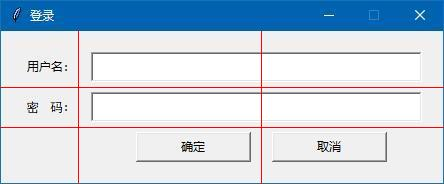语法：grid( 参数….. )

row  ：组件定位表格的行

column： 组件定位表格的列

rowspan：  组件跨多少列表格框，默认1个组件占用1行1列

columnspan ：组件跨多少行表格框，默认1个组件占用1行1列

sticky ：当表格框大小组件的大小，组件默认居中显示，那这个表格框周围的空白部分，如何分配，这就由sticky来决定。具体规定如下：

默认组件在表格框中是居中对齐显示的，但通过sticky可以设定N/S/W/E 即上/下/左/右 对齐，N/S/W/E 也可以组件使用，如：

sticky=N+S  拉高组件，让组件上下填充到表格框的顶端和底端。

sticky=N+S+E  拉高组件，让组件上下填充到表格框的顶端和底端，同时，让组件靠右对齐。

sticky=N+W+W+E  拉高并拉长组件，让组件填充满一个表格框。

其它的，以此类推……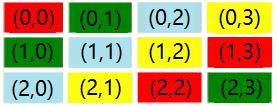### 用实例来演示grid()方法各参数的运用

```from tkinter import *
root=Tk()

la1=Label(root,text='用户名：')
la1.grid(row=0,column=0) # 0行0列

en1=Entry(root)  # 用户名文本框
en1.grid(row=0,column=1,columnspan=2) # 0行1列，跨2列

la2=Label(root,text='密　码：')
la2.grid(row=1,column=0)

en2=Entry(root)  # 密码文本框
en2.grid(row=1,column=1,columnspan=2) # 1行1列，跨2列

but1=Button(root,text="确定")
but1.grid(row=2,column=1)
but2=Button(root,text="取消")
but2.grid(row=2,column=2)

root.mainloop()```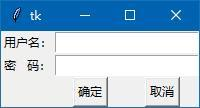```from tkinter import *
root=Tk()

la1=Label(root,text='用户名：')

en1=Entry(root)  # 用户名文本框

la2=Label(root,text='密　码：')

en2=Entry(root)  # 密码文本框

but1=Button(root,text="确定")
but2=Button(root,text="取消")

root.mainloop()```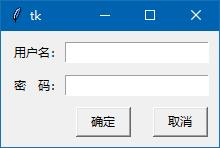```from tkinter import *
root=Tk()

la1=Label(root,text='用户名：')

en1=Entry(root)  # 用户名文本框

la2=Label(root,text='密　码：')

en2=Entry(root)  # 密码文本框

but1=Button(root,text="确定")
but2=Button(root,text="取消")

root.mainloop()```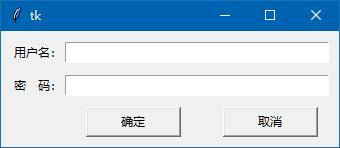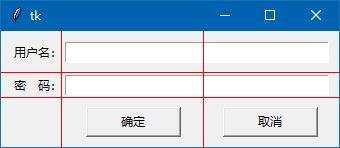```from tkinter import *
root=Tk()
root.title('登录')

la1=Label(root,text='用户名：')

en1=Entry(root)  # 用户名文本框

la2=Label(root,text='密　码：')

en2=Entry(root)  # 密码文本框

but1=Button(root,text="确定")
but2=Button(root,text="取消")

root.resizable(False,False)
root.mainloop()```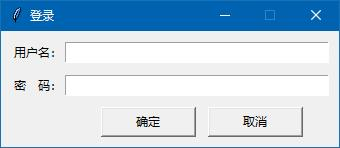```but1=Button(root,text="确定")
but2=Button(root,text="取消")

### rowconfigure() 和 columnconfigure()

rowconfigure(表格框的行，缩放权重)

columnconfigure(表格框的列，缩放权重)

rowconfigure(1, weight=1)  row为1的行(即第2行)，缩放权重为1

columnconfigure(0, weight=1) column为0的列(第1列)，缩放权重为1

columnconfigure(0, weight=1)

columnconfigure(1, weight=2)

sticky=N+S+W+E  组件的高和宽跟随表格框一起变化

sticky=N+S       组件的高跟随表格框一起变化

sticky=W+E  组件的宽跟随表格框一起变化

```from tkinter import *
root=Tk()

root.grid_rowconfigure(1,weight=1) # row为1，缩放比为1
root.grid_columnconfigure(0,weight=1) # column为0，缩放比为1

but1=Button(root,text="测试按钮1")
but1.grid(row=0,column=0)
but2=Button(root,text="测试按钮2")
but2.grid(row=0,column=1)
but3=Button(root,text="测试按钮3")
but3.grid(row=1,column=0)
but4=Button(root,text="测试按钮4")
but4.grid(row=1,column=1)
but5=Button(root,text="测试按钮5")
but5.grid(row=2,column=0,columnspan=2) # 跨2列

root.mainloop()```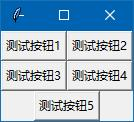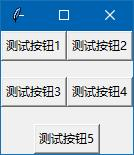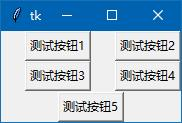```from tkinter import *
root=Tk()

root.grid_rowconfigure(1,weight=1) # row为1，缩放比为1
root.grid_columnconfigure(0,weight=1) # column为0，缩放比为1

but1=Button(root,text="测试按钮1")
but1.grid(row=0,column=0,sticky=N+S+W+E)
but2=Button(root,text="测试按钮2")
but2.grid(row=0,column=1,sticky=N+S+W+E)
but3=Button(root,text="测试按钮3")
but3.grid(row=1,column=0,sticky=N+S+W+E)
but4=Button(root,text="测试按钮4")
but4.grid(row=1,column=1,sticky=N+S+W+E)
but5=Button(root,text="测试按钮5")
but5.grid(row=2,column=0,columnspan=2,sticky=N+S+W+E) # 跨2列

root.mainloop()```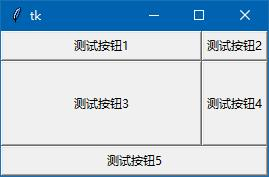### minsize 参数

有时我们希望某1行或某1列的表格框，能有一个最小的尺寸，表格框最小也不能小于这个尺寸（单位像素），我可以用以用minsize参数，如上面的代码，我们将3，4行代码修改一下，加入minsize=30 和 minsize=66

```root.grid_rowconfigure(1,weight=1,minsize=30) # 限制最小高度为30像素
root.grid_columnconfigure(0,weight=1,minsize=66) # 限制最小宽度为66像素```

大家自行运行上面的代码，把窗体尺寸变小一些，体会一下限制表格框宽度和高度最小尺寸的情况。

gride布局定位方法就讲解到这里，编程时，大家根据具体情况决定选择用pack()还是用grid()，但是这2个方法在同一个父组件内不要混用，否则运行时，系统可能卡死，我没有试过，你想试吗？

下一篇文章讲解，第3种布局定位方法 place ，虽然用得少，但也有必要了解一下，它还是有点用处的。

<< 上一篇 下一篇 >>